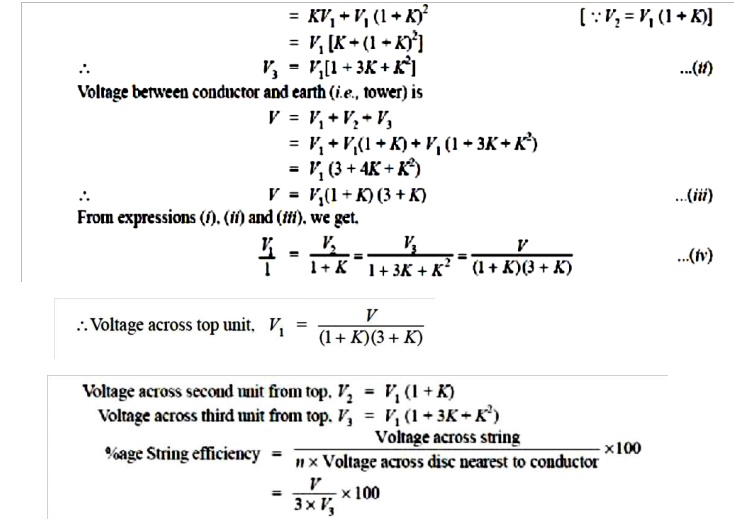Home | | Transmission and Distribution | String Efficiency

# String Efficiency

As stated above, the voltage applied across the string of suspension insulators is not uniformly distributed across various units or discs.

STRING EFFICIENCY

As  stated  above,  the  voltage  applied     across  the  string  of  suspension  insulators  is not uniformly distributed across various units or discs. The disc nearest to the conductor has much higher potential than the other discs. This unequal potential distribution is undesirable and is usually expressed in terms of string efficiency.

The ratio of voltage across the whole string to the product of number of discs and the voltage across the disc nearest to the conductor is known as string efficiency i.e.,String efficiency is an important consideration since it decides the potential distribution along the string. The greater the string efficiency, the more uniform is the voltage distribution. Thus 100% string efficiency is an ideal case for which the voltage across each disc will be exactly the same. Although it is impossible to achieve 100% string efficiency, yet efforts should be made to improve it as close to this value as possible.

Mathematical Expression. Fig. Shows the equivalent circuit for a 3-disc string. Let us suppose that self capacitance of each disc is C. Let us further assume that shunt capacitance C1 is some fraction K of self capacitance i.e., C1 =KC. Starting from the cross-arm or tower, the voltage across each unit is V1,V2 and V3 respectively as shown.The Following Points May Be Noted From The Above Mathematical Analysis

(i)  If K = 0·2 (Say), then from exp. (iv), we get, V2 = 1·2 V1 and V3 = 1·64 V1. This clearly shows that disc nearest to the conductor has maximum voltage across it; the voltage across other discs decreasing progressively as the cross-arm in approached.

(ii) The greater the value of K (= C1/C), the more non-uniform is the potential across the discs and lesser is the string efficiency.

(iii) The inequality in voltage distribution increases with the increase of number of discs in the string. Therefore, shorter string has more efficiency than the larger one

Study Material, Lecturing Notes, Assignment, Reference, Wiki description explanation, brief detail
Transmission and Distribution : Insulators and Cables : String Efficiency |